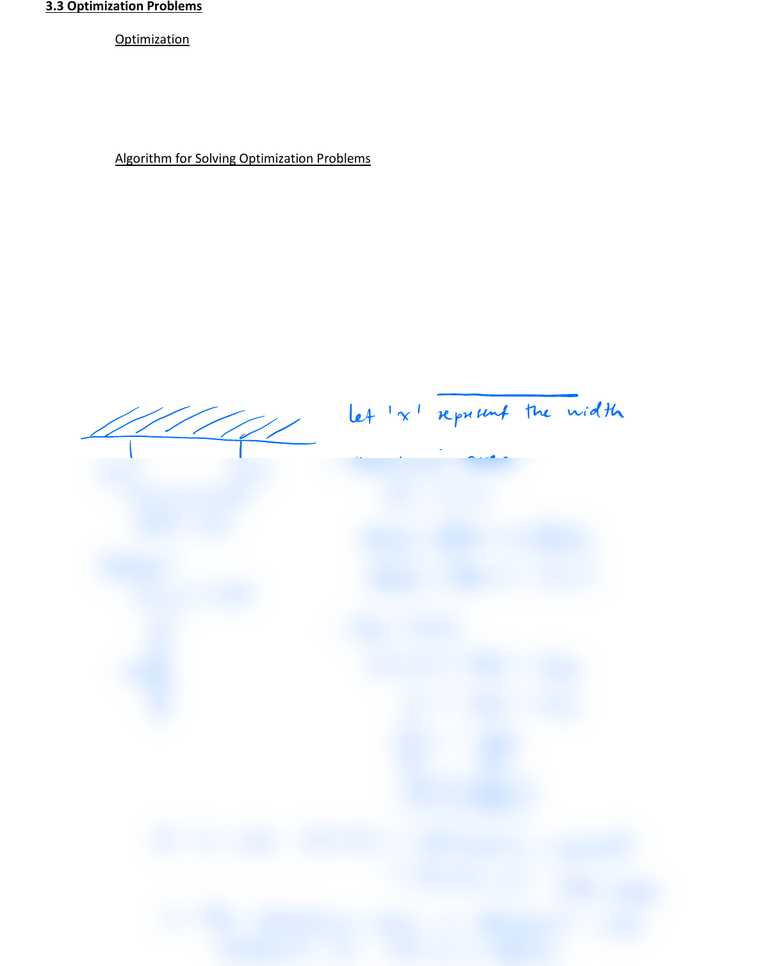# MAT135H1 Lecture 9: 3.3 Optimization Problems (3)

21 views2 pages3.3 Optimization Problems
Optimization is a procedure used in many fields to determine the best possible solution given a set of
restrictions
! Examples:
1) In engineering design (i.e. dimensions of design, cost of materials)
2) In economics (i.e. maximizing profits)
Algorithm for Solving Optimization Problems
1) Understand the problem and draw a diagram
2) Determine the quantity to be optimized
3) Create a function,
()
fx
, in one variable that represents the quantity to be optimized
[ex. dimension, price, number of units, etc]
4) Determine the domain (restrictions) of the function to be optimized
5) Use the algorithm from Section 3.2 to find the absolute maximum or minimum value in the domain
Example 1: There is 800 m of fencing available to enclose a rectangular field. One side of the field is facing a river and
does not require fencing. Determine the dimensions to enclose the maximum possible area using all of the fencing.
let x'np width
XXmaximize area
Alxw
800 2x a02x X
Domani
OLx 400 Atx 800 22
Hfu'd A'Cx
400
1Ax800 4x
bOSou 4
4X800
TF
121 200 7
at xno Ano 800 zoo zzoo
so 000 mMax area
oThe maximum area is 80000m2 with
dimension of 200 MXboom
Unlock document

This preview shows half of the first page of the document.
Unlock all 2 pages and 3 million more documents.